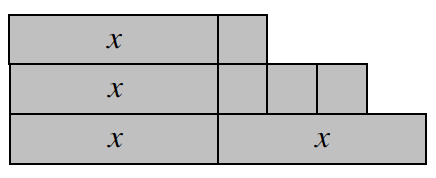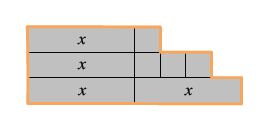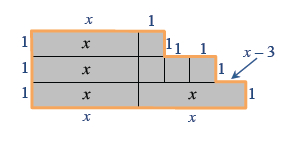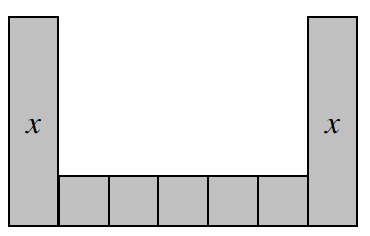Home > CC2MN > Chapter cc26 > Lesson cc26.1.3 > Problem6-34

6-34.

Find the perimeter and area of each algebra tile shape below. Be sure to combine like terms. Homework Help ✎

1.Remember, the perimeter is the sum of all the side lengths around the shape.

Labeling all the side lengths may prove to be helpful for you.

Add up all these values to find the perimeter.

The area is the space within the orange lines.

Add up the values of the algebra tiles to find the area.

$P=4x+6$
$A=4x+4$

1.See part (a).